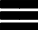Krivalar TutorialsKrivalar Tutorials

## Thread - wait() and notify() methods

Whenever we execute a main method, we will have Main Thread running. In our example, we execute a new thread invoked from the main method. New Thread is doing the calculations. The main thread needs to print the result of the output of the new thread.

This requirement can be solved by

### Example - Thread without using wait() and notify() methods

``````
int sum;
}
public void run(){
sum=0;
for(int i=0; i<1000;i++){
sum=sum+i;
}
}

public static void main(String a[]){
}
}
``````

### Output - Thread without using wait() and notify() method

```sum:0
```

In the above code, Main thread prints the results before the other thread (thread1) calculates the sum of numbers 1 to 1000. This is because the main thread (current thread) did not wait for the other thread to complete. In order to make the main thread (current thread) wait for thread1 to complete, we need to call thread1.wait() from within the main method().

``````
int sum;
}
public void run(){
sum=0;
synchronized(this){
for(int i=0; i<1000;i++){
sum=sum+i;
}
notify();
}

}

public static void main(String a[]){
try {
}
} catch (InterruptedException e) {
e.printStackTrace();
}
}
}
``````

### Output - MultiThreading with wait() and notify()

```Inside thread: Thread1
sum:499500
```